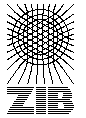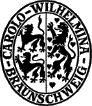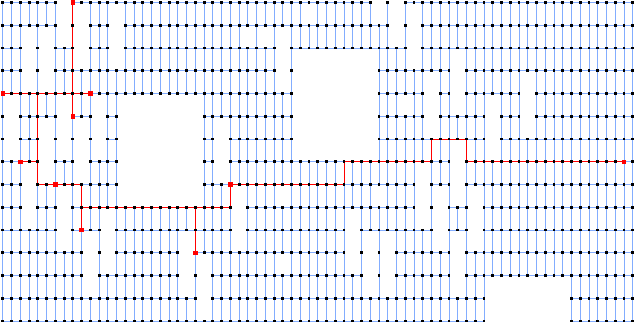# Testset ALUT

These instances are grid graphs with rectangular holes. They come from a VLSI application (see JMRW94 ).

They were introduced in KM98 . More information can be found among others in UdAR99 PD00 , and PV01c .

Here is an image of alue7080 PS DjVu with solution made by E. Uchoa .

This is a picture of diw0495 with solution.Name   |V|    |E|    |T|    DC    Opt
alut07871160 2089 34 Ps 982
alut0805966 1666 34 Ps 958
alut11813041 5693 64 Ps 2353
alut20106104 11011 68 Ps 3307
alut22889070 16595 68 NPs 3843
alut25665021 9055 68 NPs 3073
alut261033901 62816 204 Pm 12239
alut262536711 68117 879 NPm 35459
alut2764387 626 34 Ls 640

The column DC classifies the difficulty of the instance.

L
Solvable by usage of local preprocessing. Typical examples are the SD-Test, BD-n Tests and FST computations. Neither a global upper nor lower bound needs to be computed.
P
Solvable by polynomial time algorithms, like dual ascent in combination with primal heuristic, a integral LP formulation or advanced preprocessing like reduced cost criteria or the RCR-Test.
NP
No polynomial time algorithm is known. Use of an exponential time enumeration sceme like Branch-and-Bound is neccessary.

The letter after class gives an impression how long it takes to solve the problem using state-of-the-art soft- and hardware. secounds means less than a minute (this includes instances which can be solved in fractions of a second). minutes means less than an hour. hours is less than a day and days is less than a week. weeks mean it takes really a long time to solve this instance. ? means the instance is not solved or the time is not known.

If the number in the Opt column is written in italics the optimum is not known. The number given is the best know upper bound.

Last Update : 2015/02/11 11:57:20 \$ by Thorsten Koch